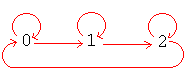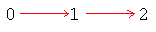# Relations represented by directed graphs

Up a level : Algebra and Arithmetic
Previous page : Classification of relations
Next page : FunctionsBinary relations represented by directed graphs

A binary relation can be represented by a directed graph. We can draw a directed links as arrows, from a to b for any two values a and b for which aRb is true. We can see this exemplified below in the examples of relations with the properties of the previous page.

The reflexivity holds if every element in a directed graph points to themselves. The graph below shows the relation “b=(a+q) mod 3 where q=0 or 1″ over the set {0,1,2}.The graph will also be an example of Antisymmetry, where the only double directed arrows are the ones possibly directed from an element onto itself.

The antireflexivity holds if no element point to itself. The graph below shows the relation a<b over the set {0,1,2}.The graph will also be an example of an asymmetry since we have no double directed relations.Up a level : Algebra and Arithmetic
Previous page : Classification of relations
Next page : FunctionsLast modified: Mar 3, 2017 @ 21:36